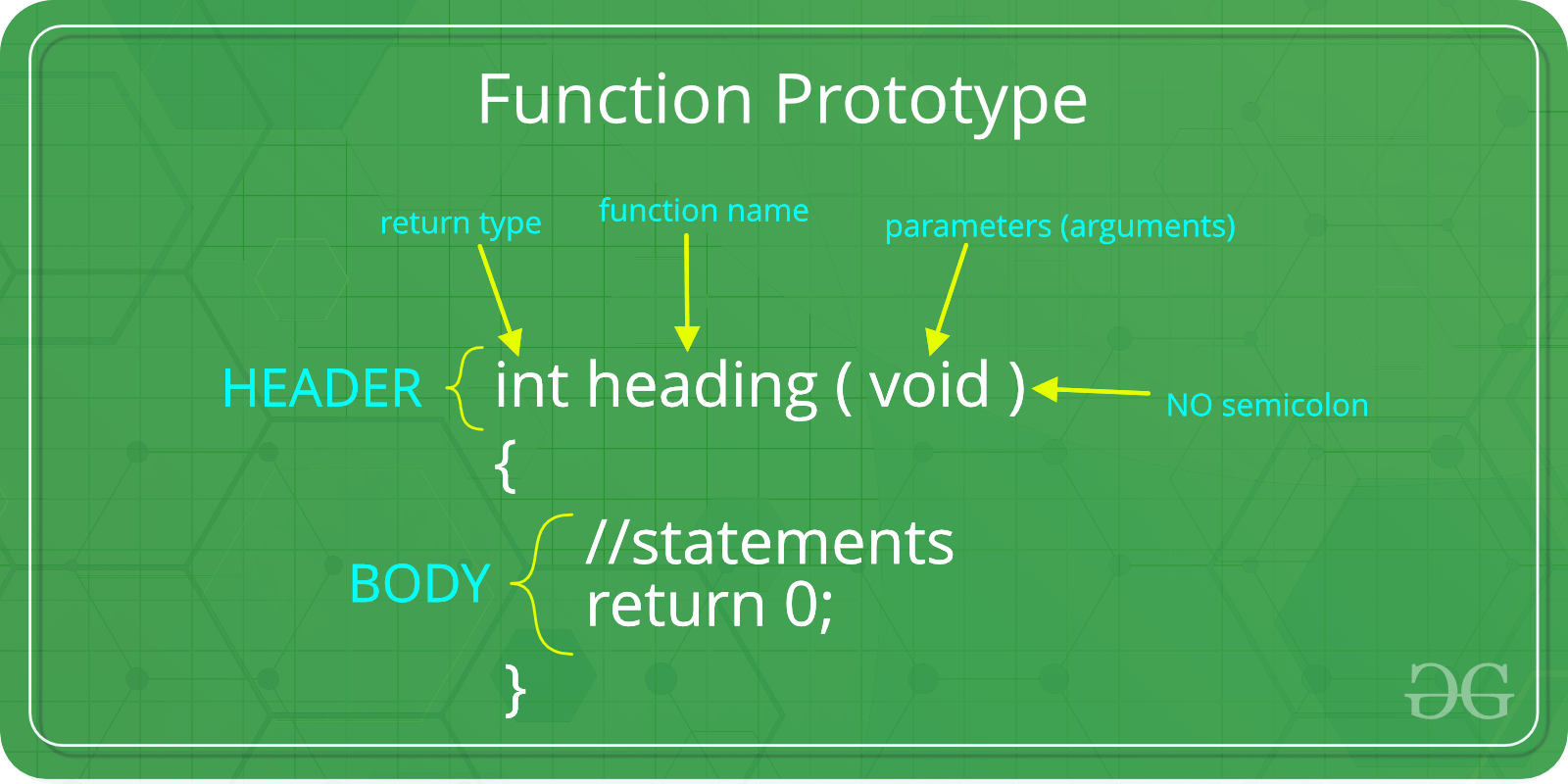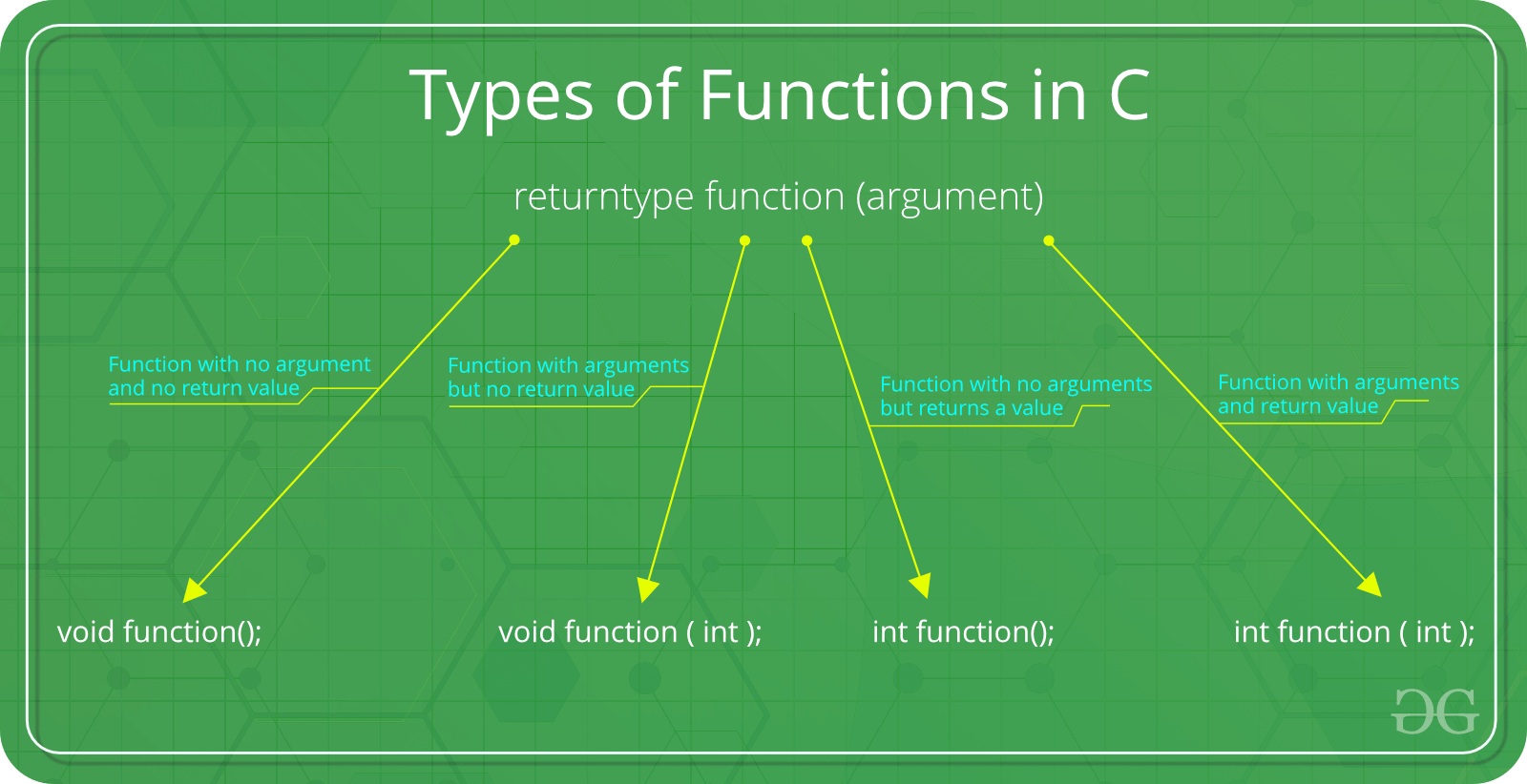# C function argument and return values

• Difficulty Level : Basic
• Last Updated : 21 Dec, 2018

Prerequisite : Functions in C/C++

A function in C can be called either with arguments or without arguments. These function may or may not return values to the calling functions. All C functions can be called either with arguments or without arguments in a C program. Also, they may or may not return any values. Hence the function prototype of a function in C is as below:Want to learn from the best curated videos and practice problems, check out the C++ Foundation Course for Basic to Advanced C++ and C++ STL Course for foundation plus STL.  To complete your preparation from learning a language to DS Algo and many more,  please refer Complete Interview Preparation Course.

There are following categories:1. Function with no argument and no return value : When a function has no arguments, it does not receive any data from the calling function. Similarly when it does not return a value, the calling function does not receive any data from the called function.
Syntax :
```Function declaration : void function();
Function call : function();
Function definition :
void function()
{
statements;
}
```
 `// C code for  function with no``//  arguments and no return value`` ` `#include ``void` `value(``void``);``void` `main()``{``    ``value();``}``void` `value(``void``)``{``    ``int` `year = 1, period = 5, amount = 5000, inrate = 0.12;``    ``float` `sum;``    ``sum = amount;``    ``while` `(year <= period) {``        ``sum = sum * (1 + inrate);``        ``year = year + 1;``    ``}``    ``printf``(``" The total amount is %f:"``, sum);``}`

Output:

```The total amount is 5000.000000
```
2. Function with arguments but no return value : When a function has arguments, it receive any data from the calling function but it returns no values.

Syntax :

```Function declaration : void function ( int );
Function call : function( x );
Function definition:
void function( int x )
{
statements;
}
```
 `// C code for function ``// with argument but no return value``#include `` ` `void` `function(``int``, ``int``[], ``char``[]);``int` `main()``{``    ``int` `a = 20;``    ``int` `ar = { 10, 20, 30, 40, 50 };``    ``char` `str = ``"geeksforgeeks"``;``    ``function(a, &ar, &str);``    ``return` `0;``}`` ` `void` `function(``int` `a, ``int``* ar, ``char``* str)``{``    ``int` `i;``    ``printf``(``"value of a is %d\n\n"``, a);``    ``for` `(i = 0; i < 5; i++) {``        ``printf``(``"value of ar[%d] is %d\n"``, i, ar[i]);``    ``}``    ``printf``(``"\nvalue of str is %s\n"``, str);``}`

Output:

```value of a is 20
value of ar is 10
value of ar is 20
value of ar is 30
value of ar is 40
value of ar is 50
The given string is : geeksforgeeks
```
3. Function with no arguments but returns a value : There could be occasions where we may need to design functions that may not take any arguments but returns a value to the calling function. A example for this is getchar function it has no parameters but it returns an integer an integer type data that represents a character.
Syntax :
```Function declaration : int function();
Function call : function();
Function definition :
int function()
{
statements;
return x;
}
```
 `// C code for function with no arguments ``// but have return value``#include ``#include `` ` `int` `sum();``int` `main()``{``    ``int` `num;``    ``num = sum();``    ``printf``(``"\nSum of two given values = %d"``, num);``    ``return` `0;``}`` ` `int` `sum()``{``    ``int` `a = 50, b = 80, sum;``    ``sum = ``sqrt``(a) + ``sqrt``(b);``    ``return` `sum;``}`

Output:

```Sum of two given values = 16
```
4. Function with arguments and return value
Syntax :
```Function declaration : int function ( int );
Function call : function( x );
Function definition:
int function( int x )
{
statements;
return x;
}
```
 `// C code for function with arguments ``// and with return value`` ` `#include ``#include ``int` `function(``int``, ``int``[]);`` ` `int` `main()``{``    ``int` `i, a = 20;``    ``int` `arr = { 10, 20, 30, 40, 50 };``    ``a = function(a, &arr);``    ``printf``(``"value of a is %d\n"``, a);``    ``for` `(i = 0; i < 5; i++) {``        ``printf``(``"value of arr[%d] is %d\n"``, i, arr[i]);``    ``}``    ``return` `0;``}`` ` `int` `function(``int` `a, ``int``* arr)``{``    ``int` `i;``    ``a = a + 20;``    ``arr = arr + 50;``    ``arr = arr + 50;``    ``arr = arr + 50;``    ``arr = arr + 50;``    ``arr = arr + 50;``    ``return` `a;``}`

Output:

```value of a is 40
value of arr is 60
value of arr is 70
value of arr is 80
value of arr is 90
value of arr is 100
```

My Personal Notes arrow_drop_up